output.to from Sideway
Draft for Information Only

# Content

```  MatLab: Basics   Arrays   Variables   Array and Variable Creation   Array Creation    Array Creation by entering elements within matrix constructor operator, [ ]     Examples    Array Creation by generating an array with elements of a numeric sequence by the colon operator, :     Syntax     Parameters     Examples    Array Creation by using the builtin specialized array function     Examples    Array Creation by concatenating arrays within matrix constructor operator, [ ]     Examples   Array Creation by using the builtin specialized array concatenation function     Examples   Variable Creation    Variable Creation by statement    Syntax    Parameters    Examples    Variable Creation from keyboard    Syntax    Parameters    Examples    Variable Creation from a file     Syntax    Parameters    Examples```

## MatLab: Basics

Major Reference Source: MatLab Verson 7.0

MatLab Package is designed for array or matrix operations, and therefore all data are stored in the form of arrays.

### Arrays

All types of data in MatLab are stored in the form of arrays. Arrays can have more than one dimension. For example

• One-dimensional array: Elements of a one-dimensional array are aligned in one single line linearly in the array. A one-dimensional array is also called a vector. Only one index variable is needed to identify each element in a one-dimensional array. For example, ai.

• A row vector is a one-dimensional array with a single row of elements where elements are aligned horizontally.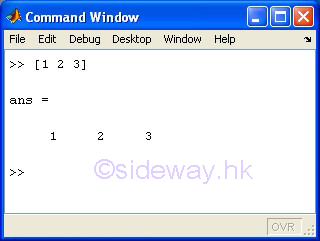• A column vector is a one-dimensional  array with a single column of elements where elements are aligned vertically.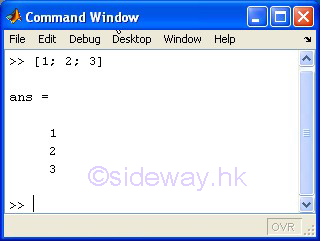• Two-dimensional array: Elements of the array is aligned in two dirmension rectangularly in the array. A two-dimensional array is also called a matrix or rectangular array. Elements of a two-dimensional array are arranged in rows and columns. And therefore a row vector is also called a row matrix and a column vector is called a column matrix. Two index variables are needed to identify each element in a two-dimensional array. For example, aij.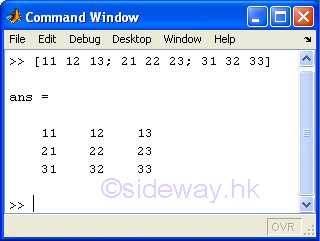• Multi-dimensional array: In general multi-dimensional array is the extension of the two-dimensional array. Similar to one- and two- dimensional array, elements of a multi-dimensional array are arranged in multi-dimensional order..

• A three-dimensional array is a three-dimensional array with one more dimension than a two-dimensional array. There index variables are needed to identify each element in a three-dimensional array. Besides the row and column dimensions, one more dimension, e.g. page, is needed to identified all elements in a three-dimensional array. For example, aijk.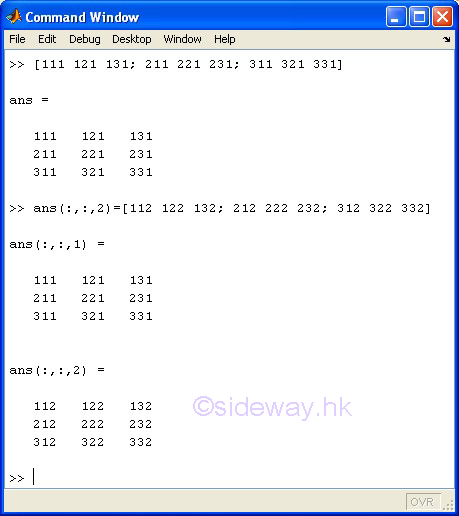The size of an array refers to the number of maximum index in each diemension not the dimension of an array. For example, the size of a matrix is given by the number of rows and columns.

### Variables

In general, a variable is used by MatLab to represent a specific piece of computer memory occupied by an array. A variable name, which can be specified by user, is given to each variable for the identification of variables stored in the memory. If no variable name is specified for a variable, a variable name, ans, is automatically created as the identification of variable stored the most recent evaluated result.

A valid MatLab variable name have the following properties:

•  A variable name can contain uppercase letters, lowercase letters, digits, or underscores character.

• MatLab variable name is case sensitive

• The MATLAB maximum effective name length of a variable name is 63 characters and variable name more than 63 characters will be truncated to 63 characters only. The value of maximum length of variable name can be obtained by the namelengthmax command.

• A variable name cannot be the same names as MatLab keysword.

### Array and Variable Creation

Square Brackets, [ ], are used to form an array, i.e. vectors, matrices and multi-dimensional arrays in MatLab.  Entering a pair of square brackets, [ ] in the Command Window is the most simplest way of creating an array. As no user-specified variable name is assigned to the created array, the variable name, ans, is automatically generated by MatLab and  is assigned to the created variable, the empty array.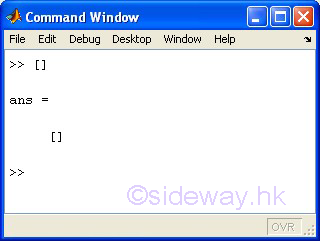### Array Creation

In general, an array is used by MatLab to represent the data structure or form for storing multiple elements of data in memory. The creation or construction of an array can be done by

• using the matrix constructor operator, [ ].

• using the colon operator, :.

• using specialized array function

• concatenating arrays with the matrix constructor operator, [ ]

• using specialized array concatenation function

#### Array Creation by entering elements within matrix constructor operator, [ ]

The simplest way to construct an array is making use of the matrix constructor operator, that is a pair of square brackets, [ ], to input elements of matrix. The open square bracket is used to indicate the begin of a matrix, while the close square bracket is used to indecate the end of the matrix. Elements of the matrix are entered within the brackets. A matrix constructor operator can only be used to construct a matrix, that is a two dimensional array. A one dimensional array can also be interpreted as two dimenstional array with one dimension equals to one. Similarly, a empty array can be interpreted as a 0-by-0 matrix and a scalar can be interpreted as a 1-by-1 matrix. Therefore a matrix constructor operator can be used to construct a empty array, a scalar, or a vector also.

Elements are entered row by row from left to right within the matrix constructor operator, [ ]. Both comma, ',' or space, ' ', can be used to separate each element within a row, while a semicolon, ';' or a newline are used to separate one row from other elements of another row within the matrix. When using space ' ' as element separator, the sign of a signed number must immediately precedes the numeric value.

##### Examples
• empty, or 0-by-0 or 1-by-0 array.• scalar or 1-by-1 array, same as entering one single element without the matrix constructor operator, [ ]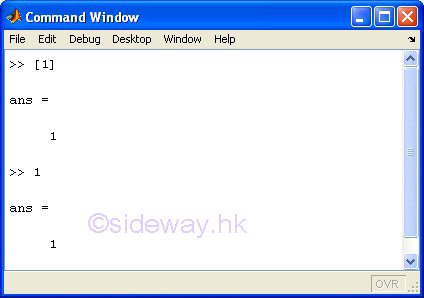• row vector or 1-by-n array with space separator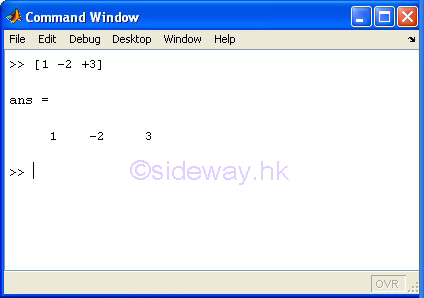•  row vector or 1-by-n array with space separator placed between the sign and the numeric value of a signed number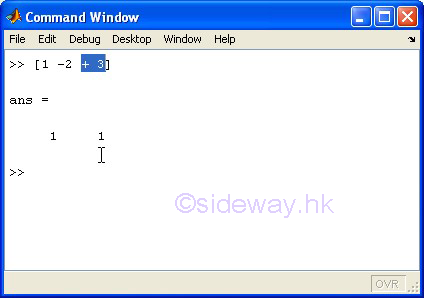• row vector or 1-by-n array with comma separator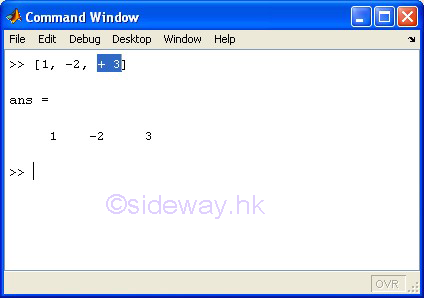• column vector or n-by-1 array with semicolon separator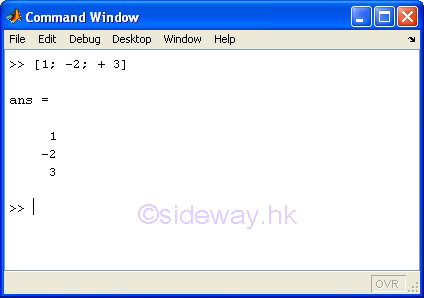• column vector or n-by-1 array with semicolon separator and newline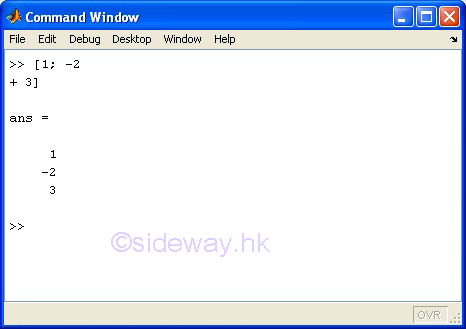• matrix., or two-dimensional array or m-by-n array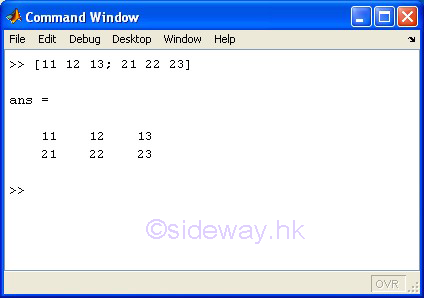#### Array Creation by generating an array with elements of a numeric sequence by the colon operator, :

The colon operator is an easy way to generate a numeric sequence in the form of a row array. Since an increasing unit-step sequence is commonly used in linear indexing and matrix construction, the simplest form of default colon operator is a simple increasing numeric sequence generator with default step +1 starting from the specified first value to the specified last value. Besides the default step of =1, a step value, either positive or negative, can also be specified.

##### Syntax

smallvalue : largetvalue

startvalue : stepvalue : lastvalue

##### Parameters

smallvalue : is to specified the start value of an increasing unit-step sequence.

largevalue : is to specified the last value of an increasing unit-step sequence.

startvalue : is to specified the start value of a numeric sequence.

stepvalue : is to specified the step of a numeric sequence.

lastvalue : is to specified the last value of a numeric sequence.

##### Examples
• to create an increasing unit-step sequence with smallvalue 5 and large value 10.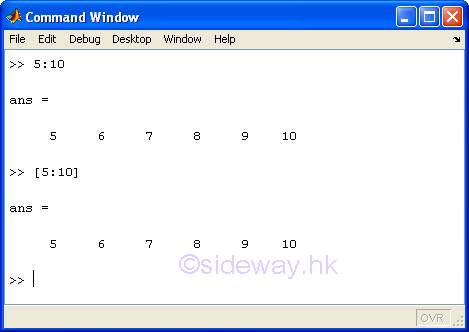• to create an increasing sequence with step value 2 from startvalue 5 to last value 13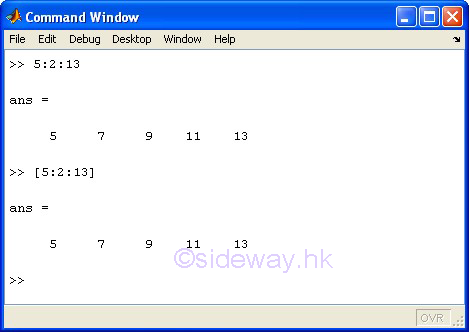• to create an decreasing sequence with step value -2 from startvalue 13 to last value 5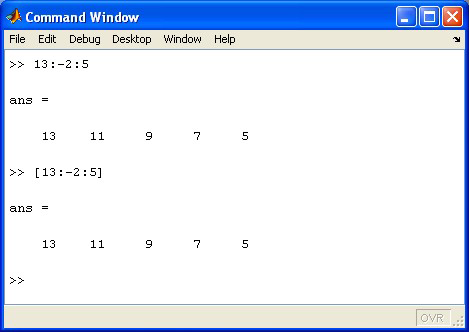#### Array Creation by using the builtin specialized array function

Since matrices or arrays can often be categorized according to the configurations of their elements or entries, MatLab provides some builtin specialized array functions that can be used to create some common kinds of matrices or arrays

##### Examples
• to create an n-by-n square matrix of 1s by ones(n) with n=3.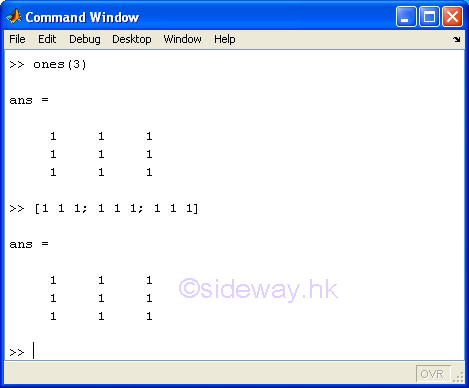• to create an m-by-n matrix of 1s by ones(m, n) or ones([m,n]) with m=3 and n=2.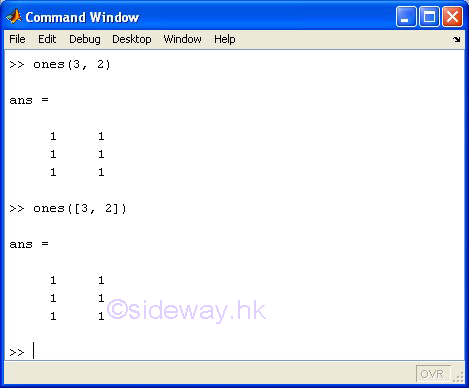• to create an m-by-n-by-o array of 1s by ones(m, n, o) or ones([m,n, o]) with m=3, n=2 and o=2.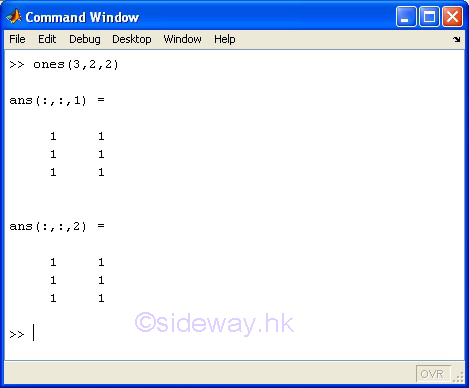• to create an n-by-n square matrix of 0s by zeros(n) with n=3.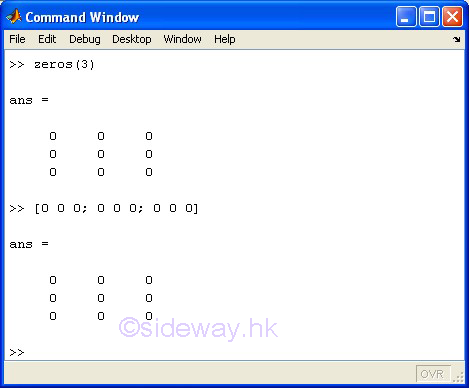• to create an m-by-n matrix of 0s by zeros(m, n) or zeros([m,n]) with m=2 and n=3.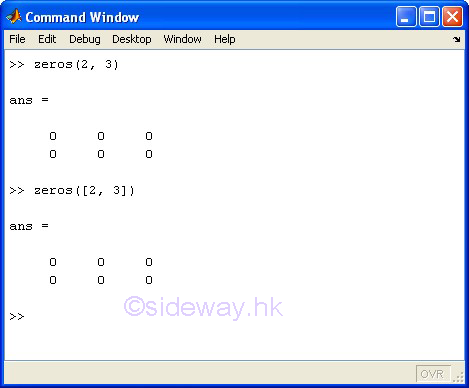• to create an m-by-n-by-o array of 1s by zeros(m, n, o) or zeros([m,n, o]) with m=3, n=2 and o=2.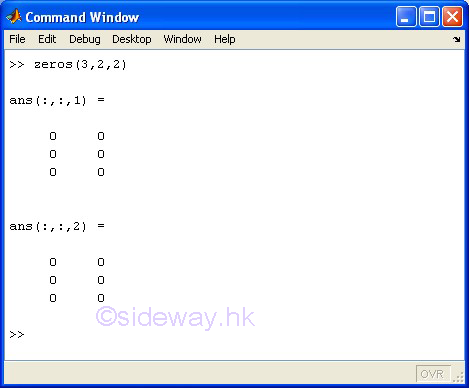• to create an n-by-n square identity matrix by eye(n) with n=3.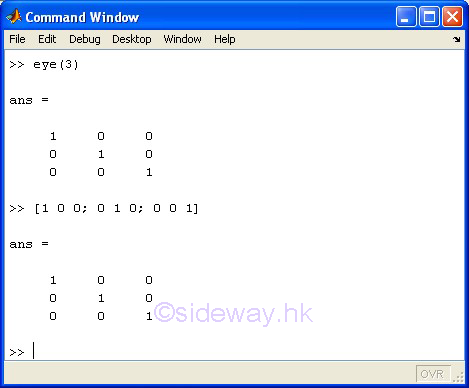• to create an m-by-n matrix with 1s on diagonal and 0s on others by eye(m,n) or eye([m,n]) with m=2 and n=3.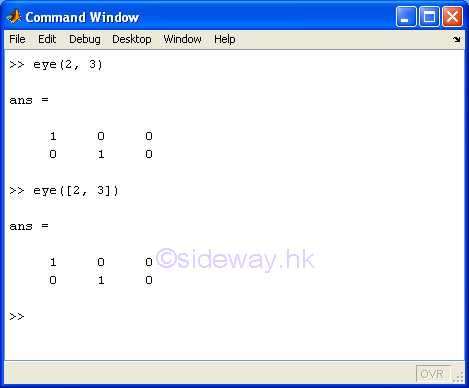• to create an m-by-n matrix with 1s on diagonal and 0s on others by eye(m,n) or eye([m,n]) with m=3 and n=2.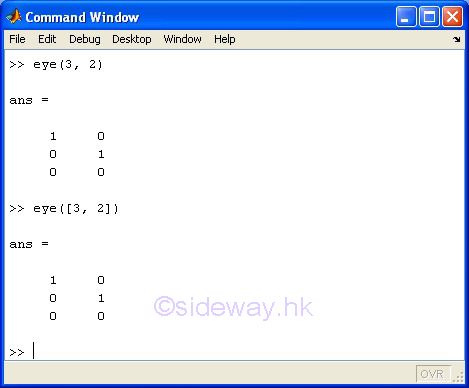• to create an n-by-n square diagonal matrix from a vector v of n components by diag(v) on the main diagonal with v=[1 2 3 4 5] and n=5.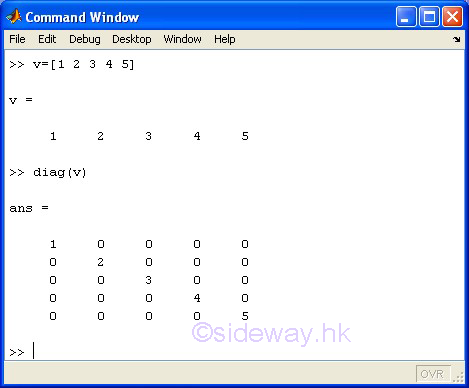• to create an m-by-m square matrix where m is equal to n+abs(k), from a vector v of n components with the vector v as a diagonal offset from the main diagonal by a distance of k, where possible k is to the right of the main diagonal and negative k is to the left of the main diagonal and 0s on others by diag(v,k) with v=[1 2 3 4 5], n=5 and k=1.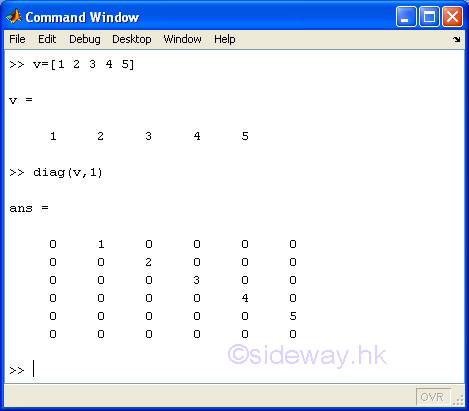• to create an n-by-n square magic matrix with the sum of elements in each column, each row or each main diagonal is the same by magic(n) with n=3.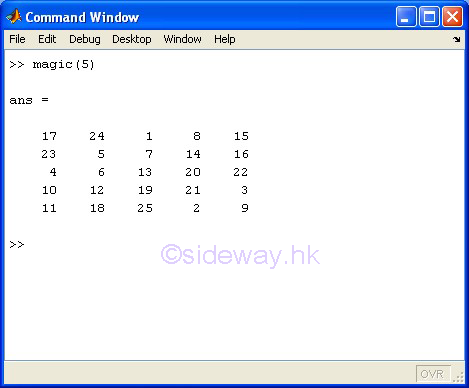#### Array Creation by concatenating arrays within matrix constructor operator, [ ]

Using the matrix constructor operator, [ ], to construct a matrix can be considered as the process of joining elements to form a matrix. The joining process of the matrix constructor operator can also be used as a matrix concatenation function to join one or more arrays to form a new array along one dimension of a matrix, that is to concatenate arrays horizontally or vertically.

##### Examples
• to concatenate arrays horizontally.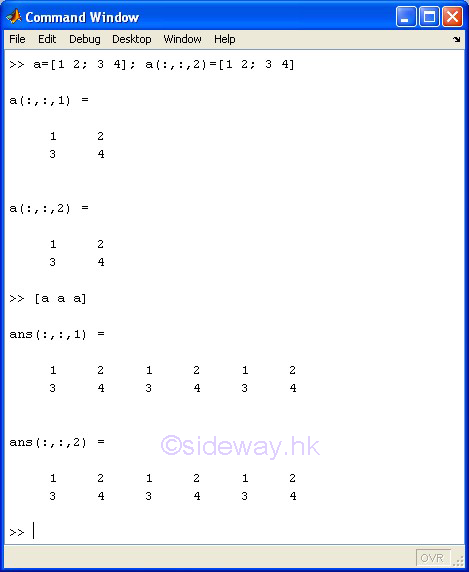• to concatenate arrays vertically.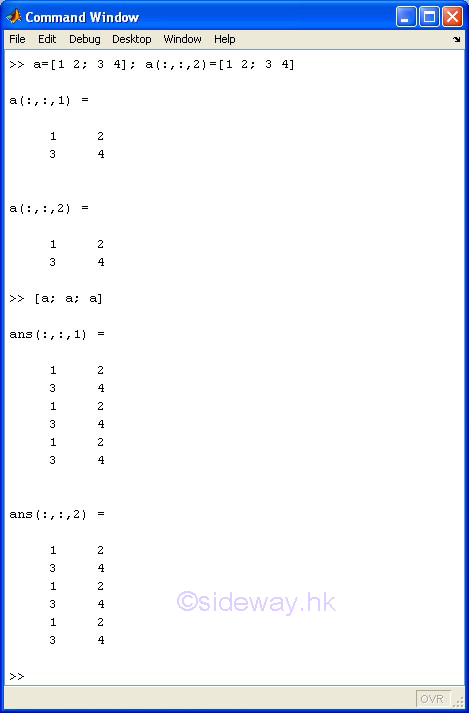### Array Creation by using the builtin specialized array concatenation function

Besides concatenating arrays within matrix constructor operator, MatLab also provides some builtin specialized array  concatenation functions that can be used to concatenate existing arrays to from a new array.

##### Examples
• to concatenate arrays, m, n, ..., horizontally by horzcat(m, n, ...) with the list of arrays are a, a, a.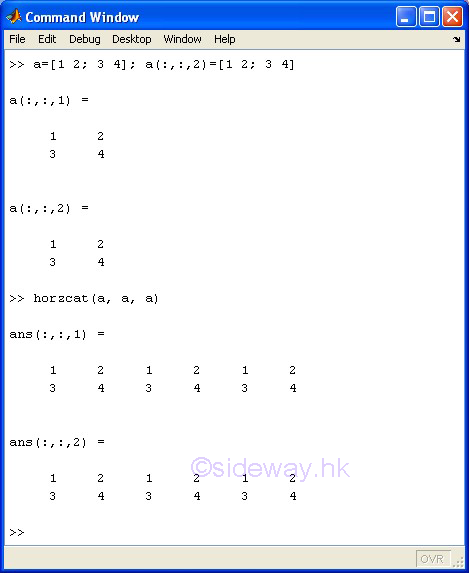• to concatenate arrays, m, n, ..., vertically by vertcat(m, n, ...) with the list of arrays are a, a, a.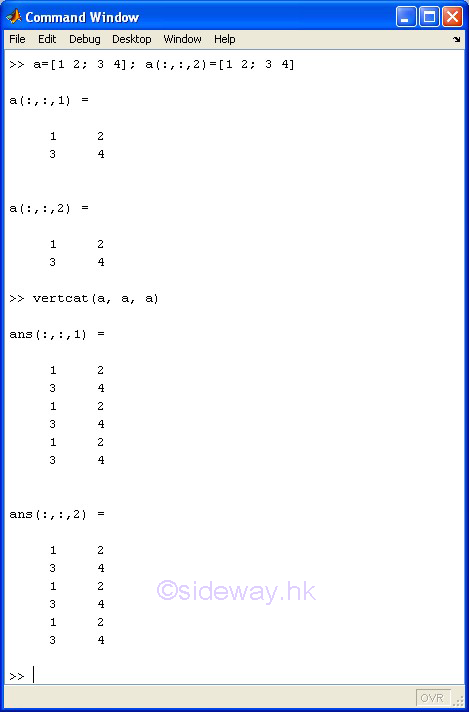• to concatenate arrays, e.g. m, n , ..., along the specified dimension, dim, by cat(dim, m, n , ...) with the list of arrays are a, a, a. and dim: 1 =  concatenate vertically, 2 = concatenate horizontally, 3 = concatenate along third dimension, n = concatenate along n dimension, etc.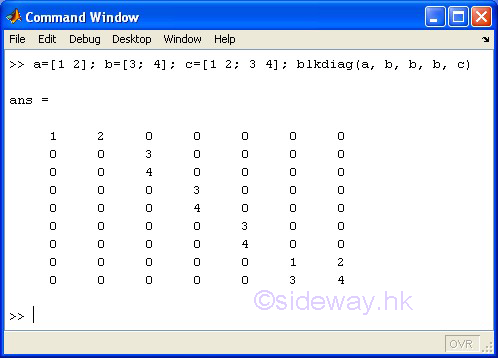• to replicate and tile an array, a, along the specified dimension, [m, n , ...], by repmat(a, [m, n , ...]) with an array a and a vector of dimension replication is [1,3,2,2].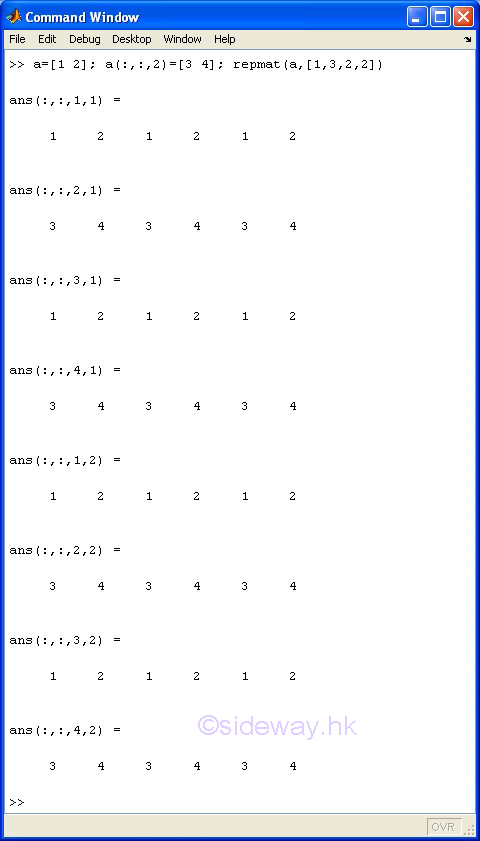• to create a matrix by placing the list of the specified arrays, m, n , ..., diagonally with others are 0s by blkdiag(m, n , ...) with the list a, b, b, b, c.### Variable Creation

User specified variables are created when these variables are initialized. Variable creation by initialization can be done by:

• an assignment statement.

• getting data from the keyboard

• retrieving all variables from a file.

#### Variable Creation by statement

An assignment statement is used to store the elements of an evaluation result of an expression to the user specified name of the array of a variable. An equal sign, =, is used as assignment in MatLab.

#### Syntax

name = expression

#### Parameters

name: is a valid MatLab variable name or named expression.

expression: is an legal MatLab expression

#### Examples

• assign an array, e.g. [1 2 3], to the user specified variable name, a.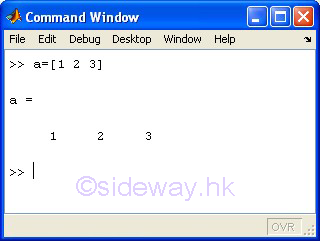• assign a sequence  of values, e.g. 1:1:3, to the user specified variable name, a.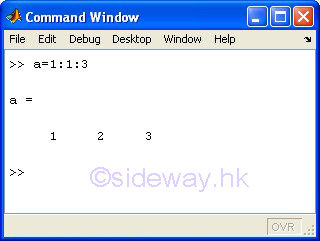• assign the varibale of variable name, a to the user specified variable name, b.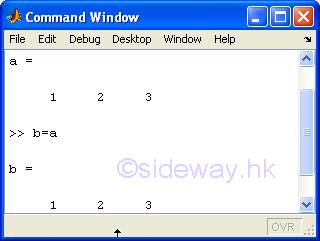• assign the evaluation result of a builtin function, e.g. ones(1,3), to the user specified variable name, a.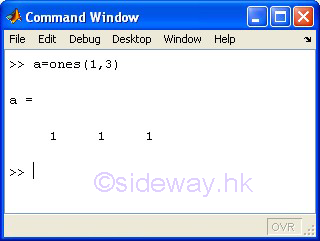#### Variable Creation from keyboard

Similar to the assignment of evaluation result of a builtin function statement, the builtin input function is used as the expression to request and get the user input by displaying an input prompt on the Command Window. Then MatLab will pause and wait for input from the keyboard. The response to the input prompt can be any legal MatLab expression as in the assignment statement. The input response can be ended by press the Return or Enter key. The input response will be evaluated first and the evaluated result is then be assigned to user_entry

#### Syntax

user_entry = input('promp'])

user_entry = input('promp'[,'s'])

#### Parameters

user_entry: is a valid MatLab variable name or named expression.

prompt: is the character string to be displayed on the screen as a prompt.

s: is an optional parameter to indicate that the entered string is interpreted as text string variable rather than as variable name or numerical value.

#### Examples

• display string "input data" to prompt user to enter data, e.g. an array [1 2 3], and assign the evaluated result to the user specified variable name, a.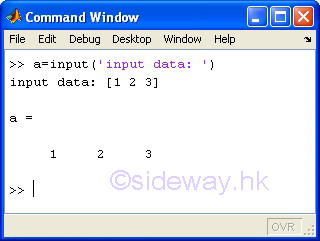• display string "input data" to prompt user to enter data, e.g.  a sequence  of values, 1:1:3, and assign the evaluated result to the user specified variable name, a.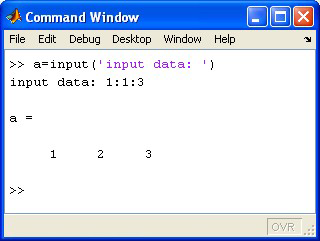• display string "input data" to prompt user to enter data, e.g.  variable name, a , and assign the evaluated result to the user specified variable name, b.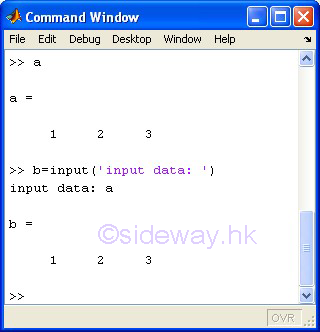• display string "input data" to prompt user to enter data, e.g.  text string, "a" , and assign the evaluated result to the user specified variable name, b.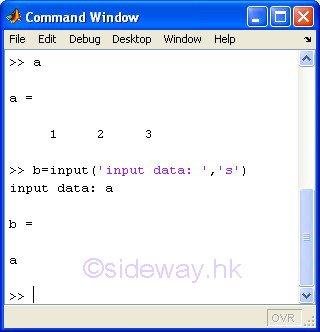• display string "input data" to prompt user to enter data, e.g. a builtin function, ones(1,3), and assign the evaluated result to the user specified variable name, a.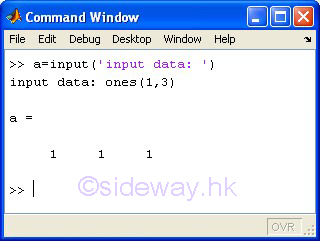#### Variable Creation from a file

A load command can also be used to load variables from the file that containing needed variables which are created and saved in the data file before. If no filename is specified, the default file is a MAT-file of file name, matlab.mat. A user specified file can also be specified if the variables are stored with other file name. The load command can also retrieive only the specified variables from the data file by appending the needed variable names with space separator to the end of load command after the file name. The file name, even using the default file name, should be specified when loading only specified variables. If no variable name is specified, all variables in the data file will be retrieved.

#### Syntax

load FILENAME X Y Z ...

#### Parameters

FILENAME: is an optional parameter to specified the data file storing the needed variables.

X Y Z ...: is the optional list of the needed variable names with space separator.

#### Examples

• load all variables in the saved default data file to the workspace.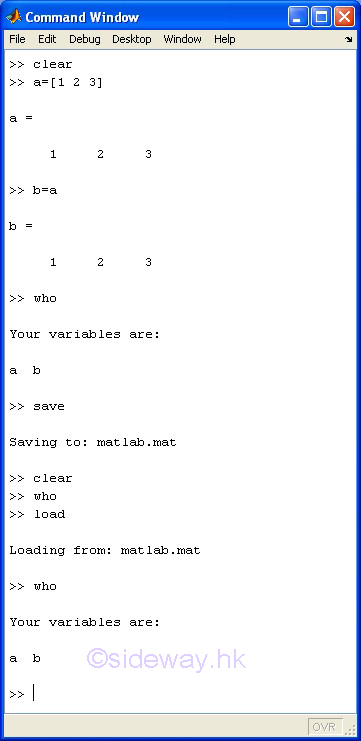• load the specified variable, e.g. a, in the saved default data file to the workspace.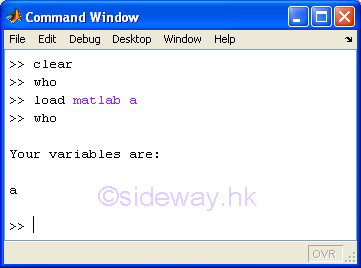ID: 140400002 Last Updated: 4/8/2014 Revision: 1Home 5

Management

HBR 3

Information

Recreation

Culture

Chinese 1097

English 339

Computer

Hardware 249

Software

Application 213

Latex 52

Manim 205

KB 1

Numeric 19

Programming

Web 289

Unicode 504

HTML 66

CSS 65

SVG 46

ASP.NET 270

OS 429

Python 72

Knowledge

Mathematics

Algebra 84

Geometry 34

Calculus 67

Engineering

Mechanical

Rigid Bodies

Statics 92

Dynamics 37

Control

Natural Sciences

Electric 27